# Test Prep Plan - Take a practice test

Take this practice test to check your existing knowledge of the course material. We'll review your answers and create a Test Prep Plan for you based on your results.
How Test Prep Plans work
1
2Based on your results, we'll create a customized Test Prep Plan just for you!
3Study smarter
Study more effectively: skip concepts you already know and focus on what you still need to learn.

# Applications of Derivatives Chapter Exam

Exam Instructions:

Choose your answers to the questions and click 'Next' to see the next set of questions. You can skip questions if you would like and come back to them later with the yellow "Go To First Skipped Question" button. When you have completed the practice exam, a green submit button will appear. Click it to see your results. Good luck!

### Page 1

#### Question 2 2. Evaluate f(1.1) by linearizing f(x) around x=1.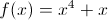#### Question 3 3. You are standing on a building on Jupiter with a ball, and you have decided that the appropriate equation of motion for the height of the ball in miles as a function of (t) is shown below on the graph. What is the highest that the ball gets?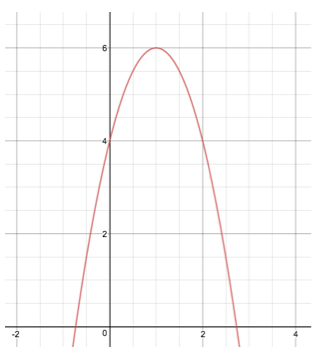#### Question 5 5. You would like to use Newton's method to find the root of f(x). What is the equation you will need to solve?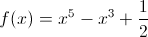### Page 2

#### Question 7 7. Linearize f(x) about the point x: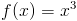#### Question 8 8. Evaluate f(2.1) by linearizing f(x) around x = 2.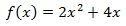#### Question 9 9. Evaluate f(pi + 0.1) by linearizing f(x) around x=pi.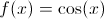#### Question 10 10. Evaluate f(2.2) by linearizing f(x) around x=2.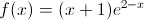### Page 3

#### Question 11 11. Where are the possible maximums and minimums located for this function?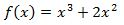#### Question 14 14. Linearize f(x) about the point x=0: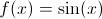### Page 4

#### Question 17 17. Linearize f(x) about the point x=0: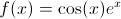#### Question 20 20. Evaluate f(1.1) by linearizing f(x) around x=1.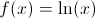### Page 6

#### Question 29 29. Linearize this function.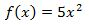#### Applications of Derivatives Chapter Exam Instructions

Choose your answers to the questions and click 'Next' to see the next set of questions. You can skip questions if you would like and come back to them later with the yellow "Go To First Skipped Question" button. When you have completed the practice exam, a green submit button will appear. Click it to see your results. Good luck!

Support